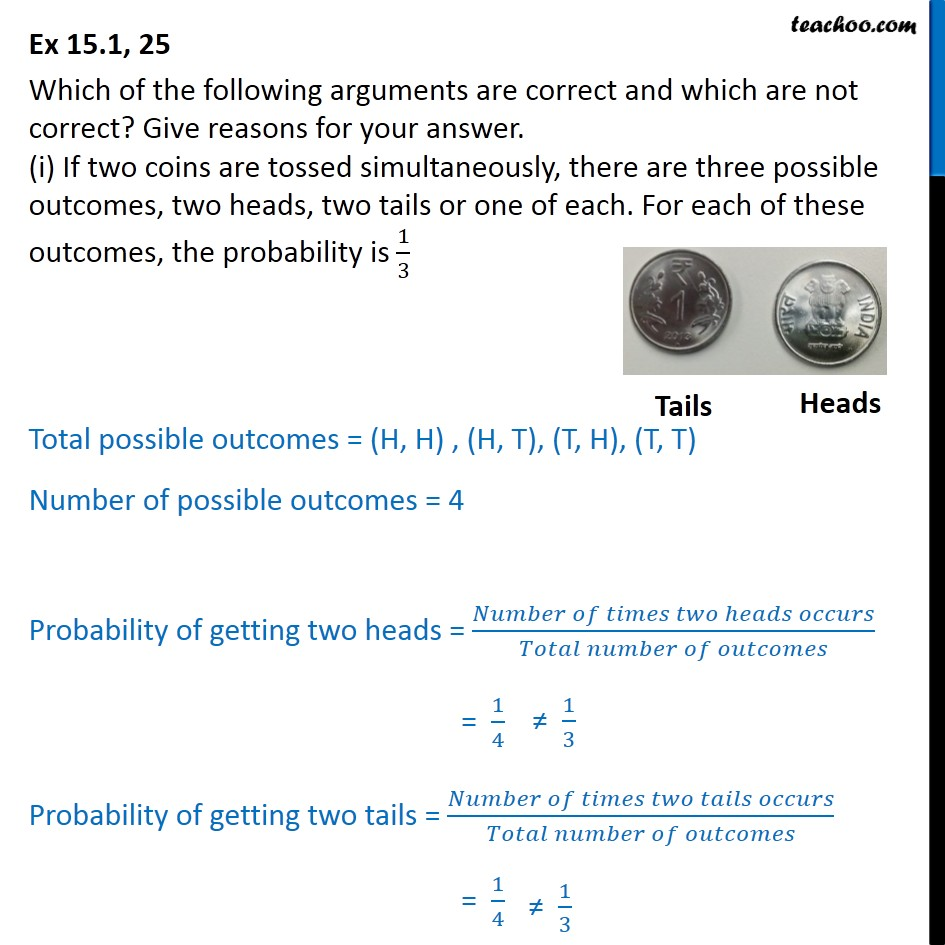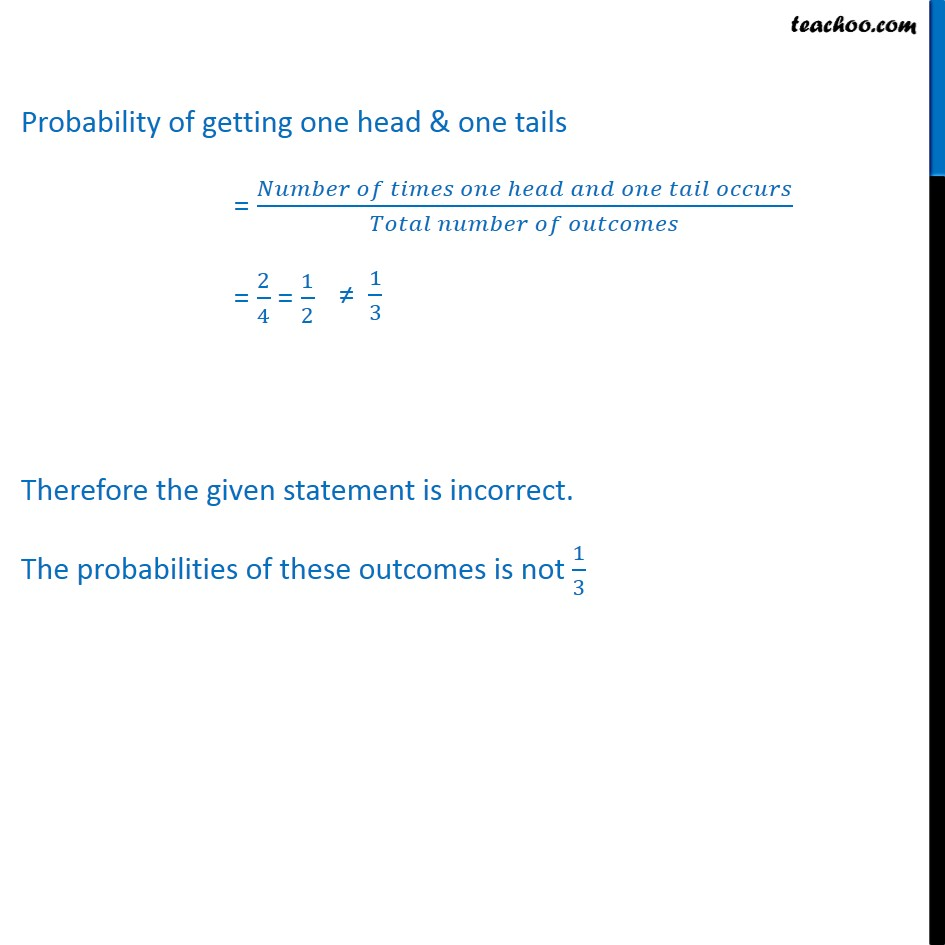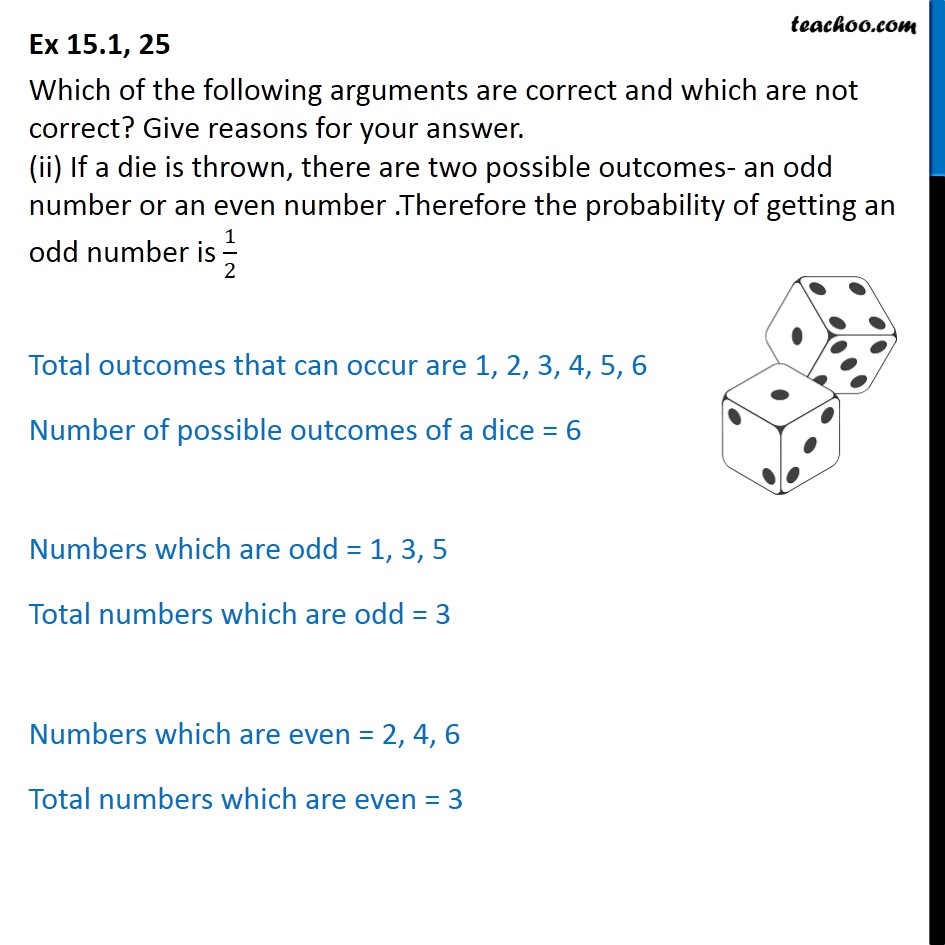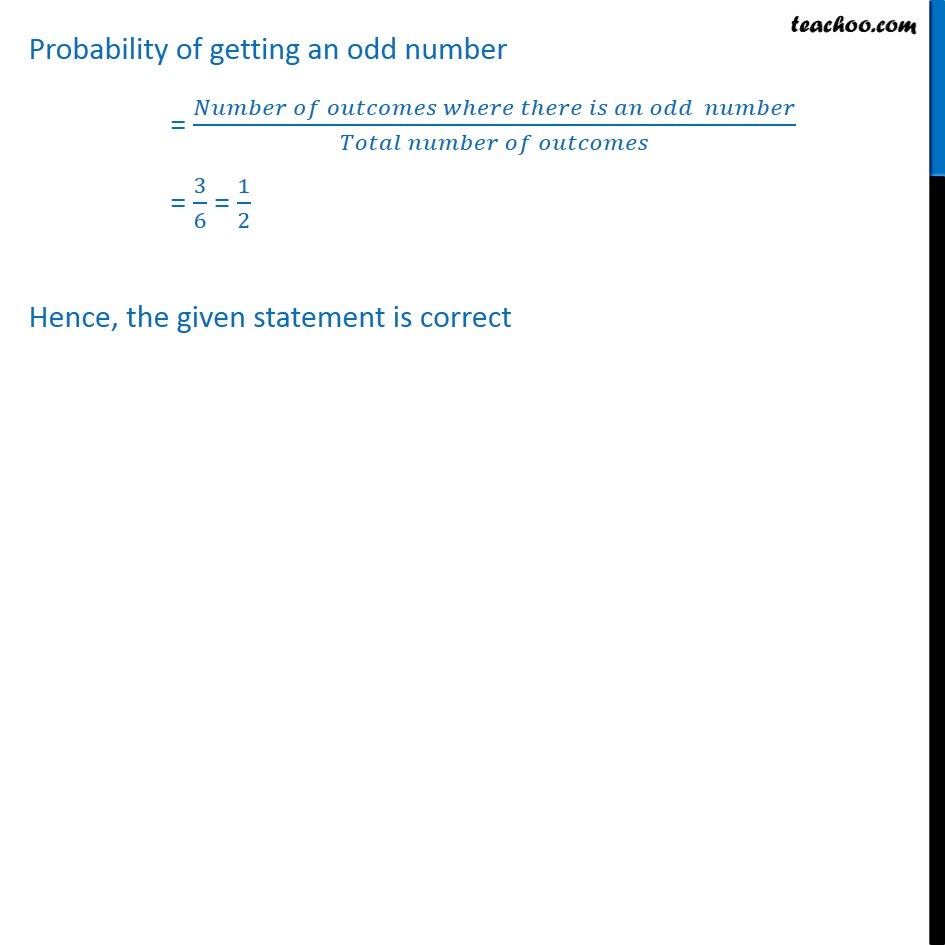Subscribe to our Youtube Channel - https://you.tube/teachoo

1. Chapter 15 Class 10 Probability
2. Serial order wise
3. Ex 15.1

Transcript

Ex 15.1, 25 Which of the following arguments are correct and which are not correct? Give reasons for your answer. (i) If two coins are tossed simultaneously, there are three possible outcomes, two heads, two tails or one of each. For each of these outcomes, the probability is 1/3 Total possible outcomes = (H, H) , (H, T), (T, H), (T, T) Number of possible outcomes = 4 Probability of getting two heads = (𝑁𝑢𝑚𝑏𝑒𝑟 𝑜𝑓 𝑡𝑖𝑚𝑒𝑠 𝑡𝑤𝑜 ℎ𝑒𝑎𝑑𝑠 𝑜𝑐𝑐𝑢𝑟𝑠)/(𝑇𝑜𝑡𝑎𝑙 𝑛𝑢𝑚𝑏𝑒𝑟 𝑜𝑓 𝑜𝑢𝑡𝑐𝑜𝑚𝑒𝑠) Probability of getting two tails = (𝑁𝑢𝑚𝑏𝑒𝑟 𝑜𝑓 𝑡𝑖𝑚𝑒𝑠 𝑡𝑤𝑜 𝑡𝑎𝑖𝑙𝑠 𝑜𝑐𝑐𝑢𝑟𝑠)/(𝑇𝑜𝑡𝑎𝑙 𝑛𝑢𝑚𝑏𝑒𝑟 𝑜𝑓 𝑜𝑢𝑡𝑐𝑜𝑚𝑒𝑠) Probability of getting one head & one tails = (𝑁𝑢𝑚𝑏𝑒𝑟 𝑜𝑓 𝑡𝑖𝑚𝑒𝑠 𝑜𝑛𝑒 ℎ𝑒𝑎𝑑 𝑎𝑛𝑑 𝑜𝑛𝑒 𝑡𝑎𝑖𝑙 𝑜𝑐𝑐𝑢𝑟𝑠)/(𝑇𝑜𝑡𝑎𝑙 𝑛𝑢𝑚𝑏𝑒𝑟 𝑜𝑓 𝑜𝑢𝑡𝑐𝑜𝑚𝑒𝑠) = 2/4 = 1/2 Therefore the given statement is incorrect. The probabilities of these outcomes is not 1/3 Ex 15.1, 25 Which of the following arguments are correct and which are not correct? Give reasons for your answer. (ii) If a die is thrown, there are two possible outcomes- an odd number or an even number .Therefore the probability of getting an odd number is 1/2 Total outcomes that can occur are 1, 2, 3, 4, 5, 6 Number of possible outcomes of a dice = 6 Numbers which are odd = 1, 3, 5 Total numbers which are odd = 3 Numbers which are even = 2, 4, 6 Total numbers which are even = 3 Probability of getting an odd number = (𝑁𝑢𝑚𝑏𝑒𝑟 𝑜𝑓 𝑜𝑢𝑡𝑐𝑜𝑚𝑒𝑠 𝑤ℎ𝑒𝑟𝑒 𝑡ℎ𝑒𝑟𝑒 𝑖𝑠 𝑎𝑛 𝑜𝑑𝑑 𝑛𝑢𝑚𝑏𝑒𝑟)/(𝑇𝑜𝑡𝑎𝑙 𝑛𝑢𝑚𝑏𝑒𝑟 𝑜𝑓 𝑜𝑢𝑡𝑐𝑜𝑚𝑒𝑠) = 3/6 = 1/2 Hence, the given statement is correct

Ex 15.1